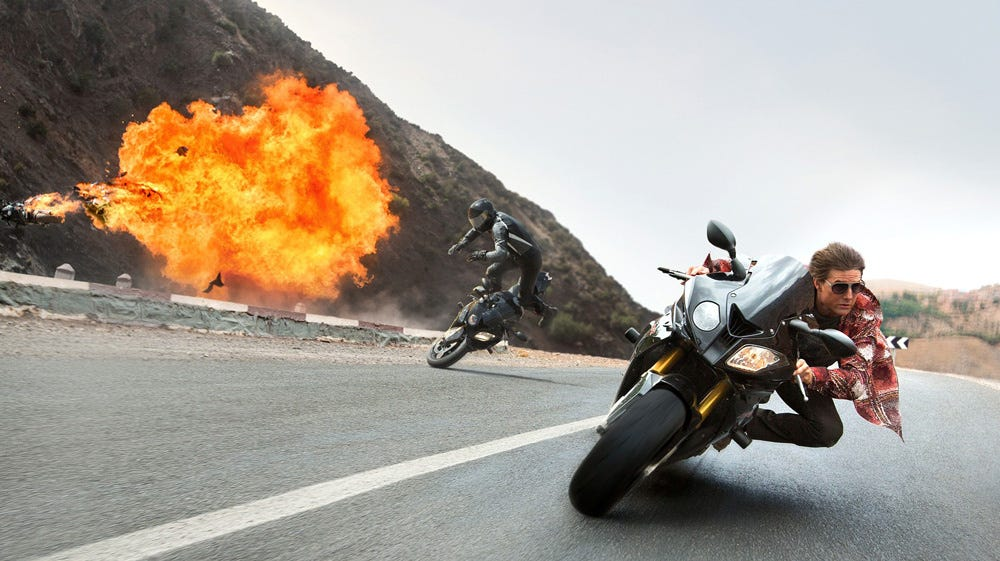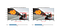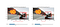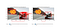# Exploring OpenCV’s Deep Learning Object Detection LibraryWill different models create different results? (Mission Impossible 6)

Deep learning for object detection on image and video has become more accessible to practitioners and programmers recently. One reason for this trend is the introduction of new software libraries, for example, TensorFlow Object Detection API, OpenCV Deep Neural Network Module, and ImageAI. These libraries have one thing in common: they all have integrated many deep-learning object-detection models into their systems. As a result, users of these libraries could reach many pre-trained models and check the best one to meet their needs. However, evaluating different models (even under the same library) might not be an easy task. This post describes a preliminary study about two deep-learning object-detection models under the Deep Neural Network Module in OpenCV 3.4.1:

• SSD/MobileNet implemented by Tensorflow, and
• YOLOv2 implemented by Darknet,

where both models are pre-trained by the COCO dataset Our focus here is to show the common workflow of using these models under OpenCV, and to explore some tricky parts in the process, such as, different operations for pre-processing images, different representations for describing bounding boxes, different formulas for calculating the confidence/probability level, etc.

Note that both SSD (stands for Single Shot MultiBox Detector) and YOLO (stands for You Only Look Once) are state-of-the-art deep-learning object-detection models. However, there are many improvements and implementations for these models. For example, researchers have used different deep neural networks (such as VGG, ResNet, or MobileNet) as feature extractor or object classifier for SSD. People have also implemented SSD under different deep learning software platforms such as Caffe, PyTorch, or Tensorflow. On the other hand, YOLO also has many variants, such as YOLOv2 and YOLOv3. Table 1 summarizes the models under OpenCV 3.4.1 for our study. Further details in the table will be discussed later.

# 1. Tom Cruise in Mission Impossible 6

Flashback to the opening scene … let’s check the detection results from SSD/MobileNet and YOLOv2 on the picture of Tom Cruise in Mission Impossible 6.Figure 1. The results of object detection from SSD/MobileNet and YOLOv2 (score = 0.40.)

It is interesting to note that different models favor different objects in this case: SSD/MobileNet detects one person and one motorcycle, while YOLOv2 detects two motorcycles.

Because both models are trained by the same dataset, we might assume that the difference could be due to the threshold value of score. Roughly speaking, the value of score indicates the confidence/probability of a detected object. The threshold value of score in Figure 1 is 0.40, where a higher score indicates higher confidence/probability of detected objects, and a lower score might result in more detected objects but with lower confidence/probability levels. Now, let’s change the threshold score to 0.30 and 0.05 then see what happens.Figure 2. The results of object detection from SSD/MobileNet and YOLOv2 (score = 0.30.)Figure 3. The results of object detection from SSD/MobileNet and YOLOv2 (score = 0.05.)

By comparing and contracting Figure 2 and Figure 3, it seems to me that

• SSD/MobileNet is an aggressive object detector, which might detect more objects but make some mistakes (pizza and donut in the picture?!)
• YOLOv2 is a conservative object detector, which might detect few objects and drop some good candidates (missing Tom Curise?!)

I have no proof to backup the above claim. Anyone? Please check more examples at the end of this post.

# 2. Workflow of Object Detection in OpenCV Deep Neural Network Module

Figure 4 shows the key workflow of using SSD/MobileNet, and Figure 5 shows the key workflow of using YOLOv2, respectively. Here is a brief on the common framework and the differences between these two models.

1. select and define the model: This step is done by in Line 4 of Figure 4, and in Line 4 of Figure 5; Note: the configuration files are downloaded from the official website.
2. pre-process the image: This step is done by in Line 6 of Figure 4 and in Line 6 of Figure 5, where is used to perform a set of pre-processing operations, such as, scaling, normalization, mean subtraction, etc. However, I feel that the parameters of this function for different models is not well documented. Please check the references at the end of this post for more details.
3. conduct the prediction: This step is done by in Line 8 of Figure 4 and in Line 8 of Figure 5; Note: this function is used to get the information of predicted objects, such as, class index, bounding boxes, confidence/probability, etc.

After executing the codes in Figure 4 and Figure 5, we will get the following shape of prediction.

• SSD/MobileNet prediction shape = (1, 1, 100, 7)
• YOLOv2 prediction shape = (845, 85)

Now, our question is why SSD/MobileNet and YOLOv2 create such prediction structures? Furthermore, how do we transform these info into class index, bounding boxes, confidence, probability, etc.?

# 3. Prediction Processing

## (1) SSD/MobileNet prediction shape = (1, 1, 100, 7)

SSD/MobileNet predicts 100 objects on an input image. Each object is specified by three attributes: a class index, a score, and a bounding box ([left, top, right, bottom]). Figure 6 shows the code for processing these attributes and drawing the bounding boxes.

## (2) YOLOv2 prediction shape = (845, 85)

YOLOv2 predicts 845 bounding boxes on an input image. The magic number 845 comes from the following design: YOLOv2 divides up an input image into a grid of 13 by 13 cells, and each cell is responsible for predicting 5 bounding boxes. So, the total number of bounding boxes on an input image is 13 x 13 x 5 = 845. Here each object is specified by three attributes: a score, a bounding box ([x_center, y_center, width, height]), and the softmax probability over 80 classes in the COCO dataset. Figure 7 shows the code for processing these attributes and drawing the bounding boxes.

## (3) Class Index and Class Name in the COCO dataset

So far, we have examined the differences between processing the prediction results under SSD/MobileNet and YOLOv2. There is one more ugly fact here. Although these two models are trained by the same COCO dataset, they use different mapping functions on the class index and the class names.

• Figure 8 shows 80 class names which are referred by YOLOv2 implemented by Darknet.
• Figure 9 shows 90 class names (with some “unknown” items) which are referred by SSD/MobileNet implemented by Tensorflow.

The problem here is because the COCO dataset puts 80 class names in 90 class index. Please see the details in here.

# 4. Conclusions

This post has described a preliminary study about the mechanisms of the deep learning object detection library in OpenCV. I would say that there is no clear winner between SSD/MobileNet implemented by Tensorflow and YOLOv2 implemented by Darknet. It depends on the needs of the applications, including a clear understanding on different performance metrics and memory usages, to guide the process of model selection.

More examples for references.

Thanks for reading. If you have any comments, please feel free to drop a note.

# 5. References

• Object detection with deep learning and OpenCV [link] (OpenCV/Caffe)
• Real-time object detection with deep learning and OpenCV [link] (OpenCV/Caffe)
• Deep learning: How OpenCV’s blobFromImage works [link] (OpenCV/Caffe)
• Running Deep Learning models in OpenCV [link] (OpenCV/YOLOv2, note: the formula for calculating score in the link and in this post are different.)
• Object Detection in OpenCV [link] (OpenCV/MobileNet)
• The Modern History of Object Recognition [link]

# 6. Follow-Up

• A Checklist for Training YOLOv3 for Our Own Dataset [link]